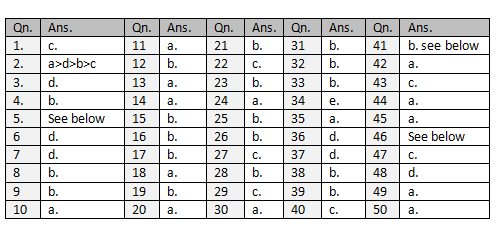# Answer Post: 50 Short Questions for Merchandisers

I posted one question set for you titled as '50 Short Questions for Merchandisers: Test Yourself where do You Stand'. I know that many of you tried to give answers to those questions and noted down those answers. As committed to you, here I am publishing answers to those questions.Question 1.
Initial quantity break-up size wise XS=100, S=200, M=300, L=200, XL=100
Size wise consumption: XS=1.0, S=1.1, M=1.2, L=1.3, XL=1.4
After changes: quantity break-up size wise S=200, M=300, L=300, XL=100
So, Size wise Fabric consumption would be -
S@1.1 * 200=220 mtrs,
M@1.2 * 300=360 mtrs,
L@1.3 * 300 = 390 mtrs
XL@1.4 * 100 =140 mtrs

Therefore average consumption =(220+360+390+140)/900 =1.23333 Meters.

Question 2.
When you break total quantity as per ratio you will size-wise quantity as following.
As a general rule size-wise consumption XS<S<M<L<XL.
So higher the quantity in higher sizes higher would be the consumption.
XS S M L XL
a. 0       1000 2000 2000 1000
b. 1000 1000 2000 2000 0
c. 1000 2000 2000 1000 0
d. 0       2000 2000 1000 1000

From the above quantity break-up, the descending order would be a<d<b<c

Question. 4.
Break total FOB Rs 400 as per cost ratio
12 :1 :3 :1 :3
240 :20 :60 :20 :60

Fabric cost increased by 5% i.e Rs. 12. So margin will be less by Rs. 12.

Current ratio is 252:20:60:20:48
Current margin = Rs. 48.
So reduction in margin = 12/60*100 = 20%

Question 5
Matching left and right hand column
a) – iv);
b) – i);
c) –v);
d) – ii);
e) – iii)

Question 41.
b. is most appropriate. Different agents used different calculation factors. It also depends on the importing country. For more reading check here.

Question 46.